Tip: Other languages are Google-Translated. You can visit the English version of this link.orRegisteror

## Excel MINUTE function

MINUTE function is common for helping to extract minute number from time cells in our Excel daily work. This article provides syntax and detailed sample of MINUTE function to help you easily use it in Excel.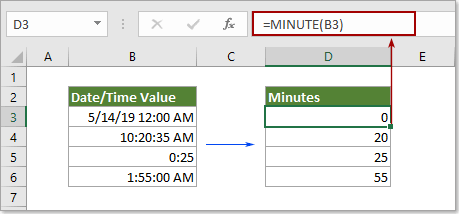Description of MINUTE function

Syntax of MINUTE function

Arguments of syntax

Example of MINUTE function

#### Description of MINUTE function

The Microsoft Excel MINUTE function extracts the minute from a time value and displays as number from 0 to 59.

#### Syntax of MINUTE function

=MINUTE (serial_number)

#### Arguments of syntax

• Serial_number: should be the valid time or date time cell or value that contains the minute you will find and extract.

1) Directly reference to a cell that contains the time or date time: =MINUTE(B3).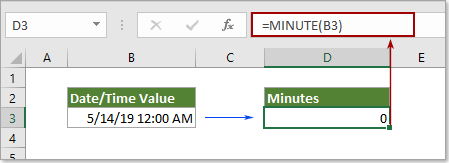2) Directly enter time as text string and embraced with quotation marks: =MINUTE("1:55 PM").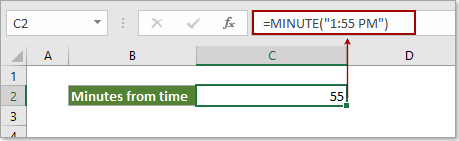3) Directly enter time as decimal numbers: =MINUTE(0.4375) (0.4375 represents the time 10:30 AM).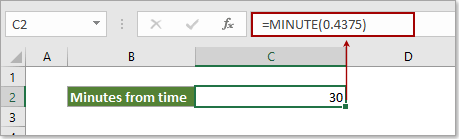4) Directly enter time as the result of a formula: =MINUTE(TIMEVALUE("10:30 AM")).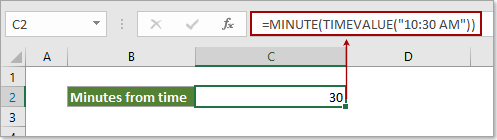#### Example of MINUTE function

As below screenshot shown, different time formats or date time formats will cause different results when using the MINUTE function in Excel.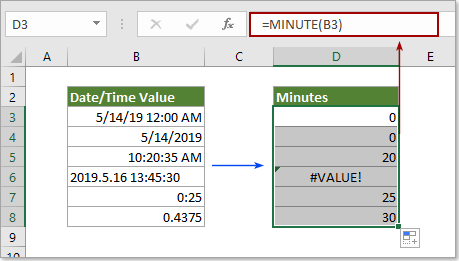Results:

 Reference Cell Data Formula Description Result 5/14/2019 12:00:00 AM =MINUTE(B3) The serial_number is a valid Date/Time format 0 5/14/2019 =MINUTE(B4) The serial_number contains no time element 0 10:20:35 AM =MINUTE(B5) The serial_number is a valid time format 20 2018.12.19 13:45:30 =MINUTE(B6) The serial_number is an invalid Date/Time format #VALUE! 0:25 =MINUTE(B7) The serial_number is corresponding decimal numbers 25 0.4375 =MINUTE(B7) The serial_number is corresponding decimal numbers 30

Remarks:

• 0 occur: occurs when the serial_number contains no time element.
• #VALUE! Occur: occurs if the serial_number is an invalid Date/Time format.Say something here...
symbols left.